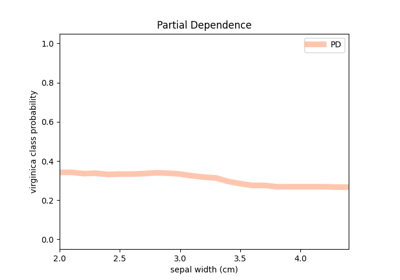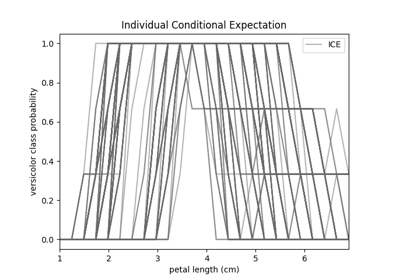# fatf.transparency.models.feature_influence.individual_conditional_expectation¶

fatf.transparency.models.feature_influence.individual_conditional_expectation(dataset: numpy.ndarray, model: object, feature_index: Union[int, str], treat_as_categorical: Optional[bool] = None, steps_number: Optional[int] = None, include_rows: Union[int, List[int], None] = None, exclude_rows: Union[int, List[int], None] = None) → Tuple[numpy.ndarray, numpy.ndarray][source]

Calculates Individual Conditional Expectation for a selected feature.

Based on the provided dataset and model this function computes Individual Conditional Expectation (ICE) of a selected feature for all target classes. If treat_as_categorical parameter is not provided the function will infer the type of the selected feature and compute the appropriate ICE. Otherwise, the user can specify whether the selected feature should be treated as a categorical or numerical feature. If the selected feature is numerical, you can specify the number of samples between this feature’s minimum and maximum value for which the input model will be evaluated. By default this value is set to 100.

Finally, it is possible to filter the rows of the input dataset that will be used to calculate ICE with include_rows and exclude_rows parameters. If include_rows is specified ICE will only be calculated for these rows. If both include and exclude parameters are given, ICE will be computed for the set difference. Finally, if only the exclude parameter is specified, these rows will be subtracted from the whole dataset.

This approach is an implementation of a method introduced by [GOLDSTEIN2015PEEKING]. It is intended to be used with probabilistic models, therefore the input model must have a predict_proba method.

GOLDSTEIN2015PEEKING

Goldstein, A., Kapelner, A., Bleich, J. and Pitkin, E., 2015. Peeking inside the black box: Visualizing statistical learning with plots of individual conditional expectation. Journal of Computational and Graphical Statistics, 24(1), pp.44-65.

Parameters
datasetnumpy.ndarray

A dataset based on which ICE will be computed.

modelobject

A fitted model which predictions will be used to calculate ICE. (Please see fatf.utils.models.models.Model class documentation for the expected model object specification.)

feature_indexUnion[integer, string]

An index of the feature column in the input dataset for which ICE will be computed.

treat_as_categoricalboolean, optional (default=None)

Whether to treat the selected feature as categorical or numerical.

steps_numberinteger, optional (default=None, i.e. 100)

The number of evenly spaced samples between the minimum and the maximum value of the selected feature for which the model’s prediction will be evaluated. (This parameter applies only to numerical features.)

include_rowsUnion[int, List[int]], optional (default=None)

Indices of rows that will be included in the ICE calculation. If this parameter is specified, ICE will only be calculated for the selected rows. If additionally exclude_rows is specified the selected rows will be a set difference between the two. This parameter can either be a list of indices or a single index (integer).

exclude_rowsUnion[int, List[int]], optional (default=None)

The indices of rows to be excluded from the ICE calculation. If this parameter is specified and include_rows is not, these indices will be excluded from all of the rows. If both include and exclude parameters are specified, the rows included in the ICE calculation will be a set difference of the two. This parameter can either be a list of indices or a single index (integer).

Returns
icenumpy.ndarray

An array of Individual Conditional Expectations for all of the selected dataset rows and the feature (dataset column) of choice. It’s of the (n_samples, steps_number, n_classes) shape where n_samples is the number of rows selected from the dataset for the ICE computation, steps_number is the number of generated samples for the selected feature and n_classes is the number of classes in the target of the dataset. The numbers in this array represent the probability of every class for every selected data point when the selected feature is fixed to one of the values in the generated feature linespace (see below).

feature_linespacenumpy.ndarray

A one-dimensional array – (steps_number, ) – with the values for which the selected feature was substituted when the dataset was evaluated with the specified model.

Raises
IncompatibleModelError

The model does not have required functionality – it needs to be able to output probabilities via predict_proba method.

IncorrectShapeError

The input dataset is not a 2-dimensional numpy array.

IndexError

Provided feature (column) index is invalid for the input dataset.

TypeError

treat_as_categorical is not None or boolean. The steps_number parameter is not None or integer. Either include_rows or exclude_rows parameter is not None, an integer or a list of integers.

ValueError

The input dataset must only contain base types (textual and numerical values). One of the include_rows or exclude_rows indices is not valid for the input dataset. The steps_number is smaller than 2.

Warns
UserWarning

The feature is treated as categorical but the number of steps parameter is provided (not None). In this case the steps_number parameter is ignored. Also, the user is warned when the selected feature is detected to be categorical (textual) while the user indicated that it is numerical.

## Examples using fatf.transparency.models.feature_influence.individual_conditional_expectation¶